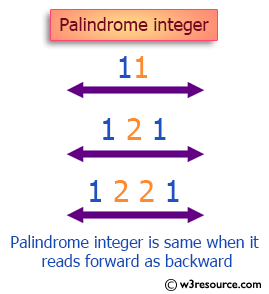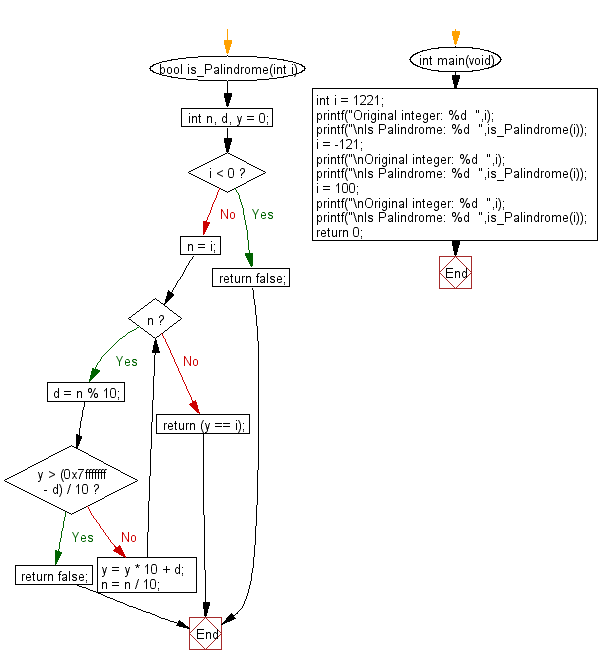﻿ C Program: Check whether an integer is a palindrome or not - w3resource

# C Exercises: Check whether an integer is a palindrome or not

## C Programming Mathematics: Exercise-2 with Solution

Write a C program to check whether an integer is a palindrome or not. An integer is a palindrome when it reads the same forward as backward.

Example:
Input:
i = 1221
i = -121
i = 100
Output:
Is Palindrome: 1
Is Palindrome: 0
Is Palindrome: 0

Pictorial Presentation:Sample Solution:

C Code:

``````#include <stdio.h>
#include <stdbool.h>

bool is_Palindrome(int i) {
int n, d, y = 0;

if (i < 0)
return false;

n = i;
while (n) {
d = n % 10;
if (y > (0x7fffffff - d) / 10)
return false;
y = y * 10 + d;
n = n / 10;
}

return (y == i);
}

int main(void)
{
int i = 1221;
printf("Original integer: %d  ",i);
printf("\nIs Palindrome: %d  ",is_Palindrome(i));
i = -121;
printf("\nOriginal integer: %d  ",i);
printf("\nIs Palindrome: %d  ",is_Palindrome(i));
i = 100;
printf("\nOriginal integer: %d  ",i);
printf("\nIs Palindrome: %d  ",is_Palindrome(i));
return 0;
}
```
```

Sample Output:

```Original integer: 1221
Is Palindrome: 1
Original integer: -121
Is Palindrome: 0
Original integer: 100
Is Palindrome: 0
```

Flowchart:C Programming Code Editor:

Improve this sample solution and post your code through Disqus.

What is the difficulty level of this exercise?

Test your Programming skills with w3resource's quiz.

﻿